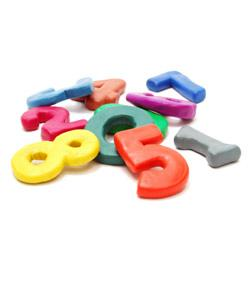# Place Value - 6 Digit Quiz

10 Questions | Attempts: 1267
ShareSettingsThis will test your knowledge around 6 digit numbers. If you successfully complete this quiz you have proven that you can:

• 1.
In 678,945 what is the value of the 6?
• A.

Tens

• B.

Thousands

• C.

Ten Thousands

• D.

Hundred Thousands

• 2.
In 217890 what is the value of the 8?
• A.

Hundred thousands

• B.

Ten thousands

• C.

Thousands

• D.

Hundreds

• 3.
In 384703 what is the value of the 4?
• A.

Thousands

• B.

Hundreds

• C.

Tens

• D.

Hundred Thousands

• 4.
Which of the following is the largest number?
• A.

234234

• B.

234284

• C.

234897

• D.

234987

• 5.
Which of the following is the largest number?
• A.

123098

• B.

123198

• C.

123298

• D.

123198

• 6.
Which of the following is the smallest number?
• A.

239487

• B.

232304

• C.

293847

• D.

203948

• 7.
Which of the following is the smallest number?
• A.

823746

• B.

879264

• C.

982734

• D.

823742

• 8.
Place the following numbers in order from smallest to largest. 239847, 234203, 234923, 897364
• 9.
Place the following numbers in order from smallest to largest. 394872, 342349, 324923, 234092
• 10.
Explain your understanding of what Place Value is and do you feel confident in working with 6 digit numbers?

## Related TopicsBack to top
×

Wait!
Here's an interesting quiz for you.